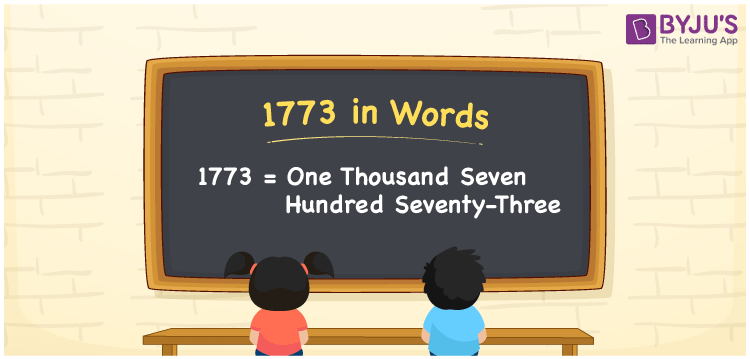# 1773 in Words

1773 in words is One thousand seven hundred seventy-three. The number 1773 is a natural number and it has four digits. The number can be converted into words easily using the place value system. Also, 1773 is a cardinal number. For example, the cost of a garment is Rs. 1773. This article discusses the spelling of 1773 in words with its procedure in detail.

 1773 in Words: One Thousand Seven Hundred Seventy-three. One Thousand Seven Hundred Seventy-three in Numerical Form: 1773.

## 1773 in English Words## How to Write 1773 in Words?

The following is the place value table for the number 1773.

 Thousands Hundreds Tens Ones 1 7 7 3

The expanded form of 1773 is as follows:

= 1 × Thousand + 7 × Hundred + 7 × Ten + 3 × One

= 1 × 1000 + 7 × 100 + 7 × 10 + 3 × 1

= 1000 + 700 + 70 + 3

= 1773

= One thousand seven hundred seventy-three

Hence, 1773 in words is one thousand seven hundred seventy-three.

The number 1773 appears after 1772 but before 1774.

1773 in words – One thousand seven hundred seventy-three

Is 1773 an odd number? – Yes

Is 1773 an even number? – No

Is 1773 a perfect square number? – No

Is 1773 a perfect cube number? – No

Is 1773 a prime number? – No

Is 1773 a composite number? – Yes

## Frequently Asked Questions on 1773 in Words

Q1

### How to spell 1773?

1773 in words is one thousand seven hundred seventy-three.

Q2

### Simplify 1700 + 73, and express it in words.

Simplifying 1700 + 73, we get 1773. Hence, 1773 in words is one thousand seven hundred seventy-three.

Q3

### Is 1773 an odd number?

Yes, 1773 is an odd number